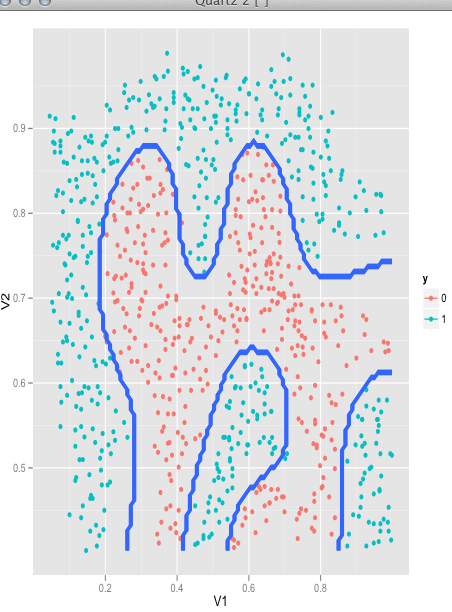SVM对于hyperplane的定义，在形式上和logistic regression一样,logistic regression的decision boundary由$\theta^TX=0$确定,SVM则用$w^TX+b=0$表示,其中b相当于logistic regression中的$\theta_0$，从形式上看，两者并无区别，当然如前面所说，两者的目标不一样，logistic regression着眼于全局，SVM着眼于support vectors。有监督算法都有label变量y，logistic regression取值是{0,1}，而SVM为了计算距离方便，取值为{-1,1}

require(mlass)
data(ex6data2)
model <- svmTrain(X,y, C=1, kernelFunction="gaussianKernel")
plot(model, X,y, type="nonlinear")